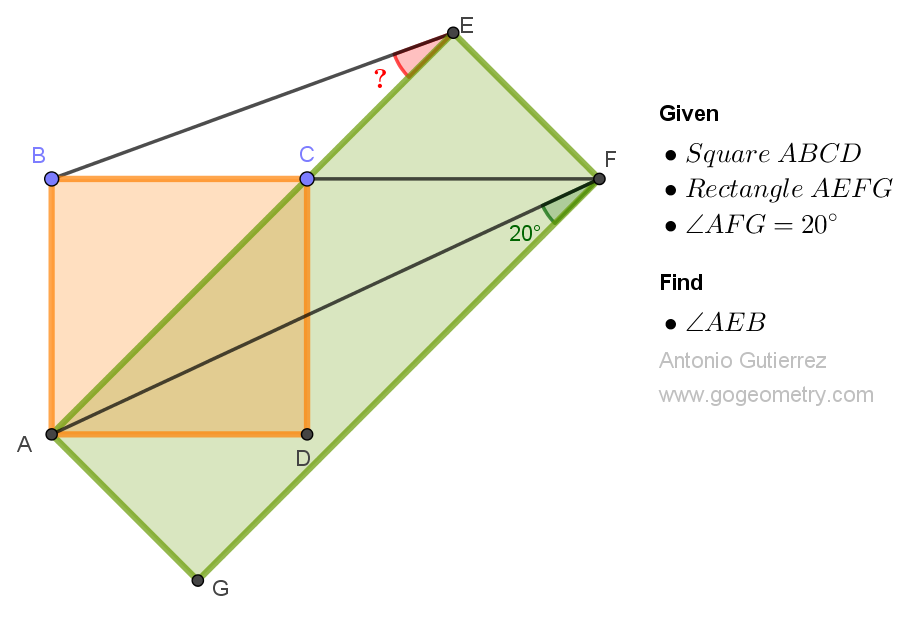# Geometry Problem 1530: Unlock the Secrets of Geometric Angles: Calculate the Measurement of an Angle in a Square and Rectangle Figure Today! - A High School Challenge

In the figure, ABCD is a square and AEFD is a rectangle such that AE and BF pass through C. If the angle AFG measures 20 degrees, calculate the measurement of the angle AEB.### Thematic Poem: Unlocking the Wonders of Geometric Angles in Squares and Rectangles

Geometry, oh geometry,
Of angles, shapes, and symmetry,
Lines and curves in harmony,
Forming figures perfectly.

In squares and rectangles, we see,
Angles that are key,
To measure them accurately,
Oh what a mystery!

But fear not, for there's a way,
To calculate and solve each day,
With formulas and tools in play,
Geometric angles will not stray.

So let us explore and learn,
To measure angles with concern,
For in geometry, we discern,
Beauty in every turn.

Unlock the secrets of angles now,
In squares and rectangles, take a bow,
For geometric wonders will endow,